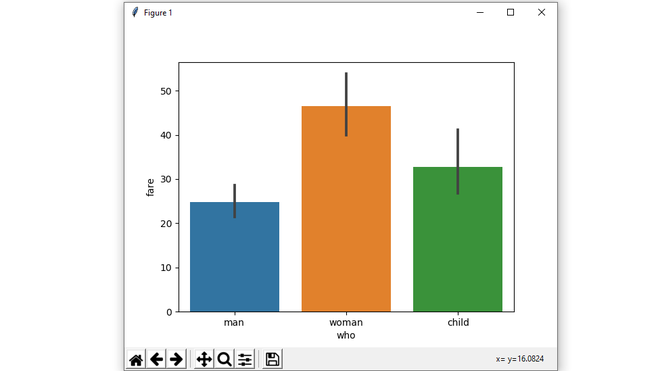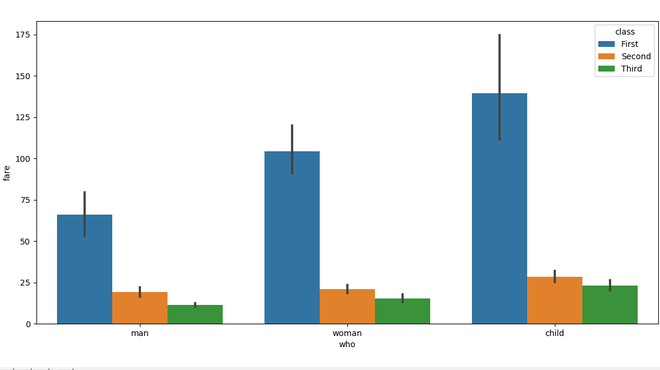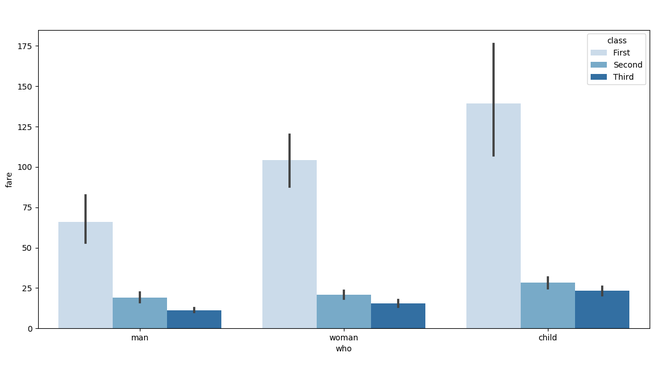# Seaborn.barplot() method in Python

## Seaborn.barplot() method in Python

Seaborn is a Python data visualization library based on Matplotlib. It provides a high-level interface for drawing attractive and informative statistical graphics. There is just something extraordinary about a well-designed visualization. The colors stand out, the layers blend nicely together, the contours flow throughout, and the overall package not only has a nice aesthetic quality, but it provides meaningful insights to us as well.

## seaborn.barplot() method

A barplot is basically used to aggregate the categorical data according to some methods and by default it’s the mean. It can also be understood as a visualization of the group by action. To use this plot we choose a categorical column for the x-axis and a numerical column for the y-axis, and we see that it creates a plot taking a mean per categorical column.

Syntax : seaborn.barplot(x=None, y=None, hue=None, data=None, order=None, hue_order=None, estimator=<function mean at 0x000002BC3EB5C4C8>, ci=95, n_boot=1000, units=None, orient=None, color=None, palette=None, saturation=0.75, errcolor=’.26′, errwidth=None, capsize=None, dodge=True, ax=None, **kwargs,)

Parameters :

Following steps are used :

• Import Seaborn
• Load Dataset from Seaborn as it contain good collection of datasets.
• Plot Bar graph using seaborn.barplot() method.

Below is the implementation :

Example 1:

 `# importing the required library` `import` `seaborn as sns` `import` `matplotlib.pyplot as plt` `# read a titanic.csv file` `# from seaborn library` `df ``=` `sns.load_dataset(``'titanic'``)` `# who v/s fare barplot` `sns.barplot(x ``=` `'who'``,` `            ``y ``=` `'fare'``,` `            ``data ``=` `df)` `# Show the plot` `plt.show()`

Output:Example 2:

 `# importing the required library` `import` `seaborn as sns` `import` `matplotlib.pyplot as plt` `# read a titanic.csv file` `# from seaborn library` `df ``=` `sns.load_dataset(``'titanic'``)` `# who v/s fare barplot` `sns.barplot(x ``=` `'who'``,` `            ``y ``=` `'fare'``,` `            ``hue ``=` `'class'``,` `            ``data ``=` `df)` `# Show the plot` `plt.show()`

Output:Example 3:

 `# importing the required library` `import` `seaborn as sns` `import` `matplotlib.pyplot as plt` `# read a titanic.csv file` `# from seaborn library` `df ``=` `sns.load_dataset(``'titanic'``)` `# who v/s fare barplot` `sns.barplot(x ``=` `'who'``,` `            ``y ``=` `'fare'``,` `            ``hue ``=` `'class'``,` `            ``data ``=` `df,` `            ``palette ``=` `"Blues"``)` `# Show the plot` `plt.show()`

Output:Example 4:

 `# importing the required library` `import` `seaborn as sns` `import` `matplotlib.pyplot as plt` `import` `numpy as np` `# read a titanic.csv file` `# from seaborn library` `df ``=` `sns.load_dataset(``'titanic'``)` `# who v/s fare barplot` `sns.barplot(x ``=` `'who'``,` `            ``y ``=` `'fare'``,` `            ``hue ``=` `'class'``,` `            ``data ``=` `df,` `            ``estimator ``=` `np.median,` `            ``ci ``=` `0``)` `# Show the plot` `plt.show()`

Output:Last Updated on March 1, 2022 by admin

## Python program to get all subsets of given size of a setPython program to get all subsets of given size of a set

Python program to get all subsets of given size of a set Given a set,

## How to Plot Mean and Standard Deviation in Pandas?How to Plot Mean and Standard Deviation in Pandas?

How to Plot Mean and Standard Deviation in Pandas? Errorbar is the plotted chart that refers

## Python – Coefficient of Determination-R2 scorePython – Coefficient of Determination-R2 score

Python – Coefficient of Determination-R2 score Coefficient of determination also called as R2 score is used

## Python program to find Cumulative sum of a listPython program to find Cumulative sum of a list

Python program to find Cumulative sum of a list The problem statement asks to produce

## Inner Class in PythonInner Class in Python

Inner Class in Python A Python is an Object-Oriented Programming Language, everything in python is related to

## Python: os.path.abspath() method with examplePython: os.path.abspath() method with example

Python: os.path.abspath() method with example OS module in Python provides various methods for interacting with the

## Billion To Rupees CalculatorBillion To Rupees Calculator

Billion to Rupees Calculator Billion: Calculate Rupees: Convert Billions to Rupees with Online Calculator Looking

## Action Chains in Selenium PythonAction Chains in Selenium Python

Action Chains in Selenium Python Selenium’s Python Module is built to perform automated testing with